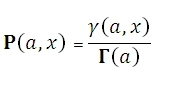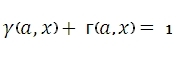# SQL Server incomplete gamma inverse distribution function

Updated: 9 August 2010

Use INVGAMMAP to calculate the inverse of the incomplete gamma function P(a,x). The equation for this function is:WhereSyntax
SELECT [wctStatistics].[wct].[INVGAMMAP] (
<@P, float,>
,<@A, float,>)
Arguments
@P
a value to be passed to the function. @P is an expression of type float or of a type that can be implicitly converted to float
@A
a value to be passed to the function. @A is an expression of type float or of a type that can be implicitly converted to float
Return Types
float
Remarks
·         If @A ≤ 0, INVGAMMAP will return an error.
·         GAMMAP(@A,INVGAMMAP(@P, @A))-@P = 0
Examples

Select
wct.INVGAMMAP(0.0526530172876507, 5)

This produces the following result
----------------------
2

(1 row(s) affected)

Select
wct.GAMMAP(5, wct.INVGAMMAP(0.0526530172876507, 5))-0.0526530172876507

This produces the following result
----------------------
0

(1 row(s) affected)Copyright 2008-2023 Westclintech LLC         Privacy Policy        Terms of Service